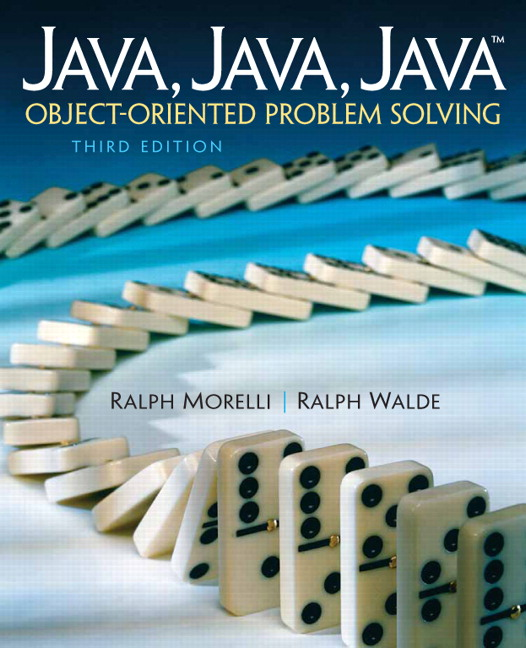# Java, Java, Java: Object-Oriented Problem Solving, 2022E

## Section10.9Chapter Exercises

1. Explain the difference between the following pairs of terms:
1. Fill in the blanks.
1. __________ an exception is Java’s way of signaling that some kind of abnormal situation has occurred.
2. The only place that an exception can be thrown in a Java program is within a __________ .
3. The block of statements placed within a catch block is generally known as an __________ .
4. To determine a statement’s __________ scope, you have to trace the program’s execution.
5. To determine a statement’s __________ scope, you can just read its definition.
6. When a method is called, a representation of the method call is placed on the __________ .
7. The root of Java’s exception hierarchy is the __________ class.
8. A __________ exception must be either caught or declared within the method in which it might be thrown. 
9. An __________ exception can be left up to Java to handle.
2. Compare and contrast the four different ways of handling exceptions within a program.
3. Suppose you have a program that asks the user to input a string of no more than five letters. Describe the steps you’d need to take in order to design a StringTooLongException to handle cases where the user types in too many characters.
4. Exceptions require more computational overhead than normal processing. Explain.
5. Suppose the following ExerciseExample program is currently executing the if statement in method2():
public class ExerciseExample {
public void method1(int M) {
try {
System.out.println("Entering try block");
method2( M );
System.out.println("Exiting try block");
} catch (Exception e) {
System.out.println("ERROR: " + e.getMessage());
}
} // method1()
public void method2(int M) {
if (M > 100)
throw new ArithmeticException(M + " is too large");
}
public static void main(String argv[]) {
ExerciseExample ex = new ExerciseExample();
ex.method1(500);
}
} // ExerciseExample

Draw a picture of the method call stack that represents this situation.
6. Repeat the previous exercise for the situation where the program is currently executing the second println() statement in method1().
7. Draw a hierarchy chart that represents the static scoping relationships among the elements of the ExerciseExample program.
8. What would be printed by the ExerciseExample program when it is run?
9. What would be printed by the ExerciseExample program, if the statement in its main method were changed to ex.method1(5)?
10. Consider again the ExerciseExample program. If the exception thrown were Exception rather than ArithmeticException, explain why we would get the following error message: java.lang.Exception must be caught, or it must be declared .
11. Write a try/catch block that throws an Exception if the value of variable X is less than zero. The exception should be an instance of Exception and, when it is caught, the message returned by getMessage() should be “ERROR: Negative value in X coordinate.”
12. Look at the IntFieldTester program (Listing 10.6.5) and the IntField class definition (Listing 10.6.4). Suppose the user inputs a value that’s greater than 100. Show what the method call stack would look like when the IntField.getInt() method is executing the num > bound expression.
13. As a continuation of the previous exercise, show what the program’s output would be if the user input a value greater than 100.
14. As a continuation of the previous exercise, modify the IntOutOfRangeException handler so that it prints the message call stack. Then show what it would print.
15. Define a subclass of RuntimeException named InvalidPasswordException, which contains two constructors. The first constructor takes no parameters and an exception thrown with this constructor should return “ERROR: invalid password” when its getMessage() is invoked. The second constructor takes a single String parameter. Exceptions thrown with this constructor should return the constructor’s argument when getMessage() is invoked.
16. Extend the IntField class so that it will constrain the integer JTextField to an int between both a lower and upper bound. In other words, it should throw an exception if the user types in a value lower than the lower bound or greater than the upper bound.
17. Design Issue: One of the preconditions for the InsertionSort() method (in Ch. 9) is that its array parameter not be null. Of course, this precondition would fail if the array were passed a null array reference. In that case, Java would throw a NullPointerException and terminate the program. Is this an appropriate way to handle that exception?
18. With respect to the previous exercise, suppose you decide that it is more appropriate to handle the NullPointerException by presenting an error dialog. Modify the method to accommodate this behavior.
19. Design Issue: Another possible way to design the sequentialSearch() method (in Ch. 9) would be to have it throw an exception when its key is not found in the array. Is this a good design? Explain.